Last Updated: 25th December, 2015# GATE Virtual Calculator Complete Tutorial

From this year GATE committee has prohibited the use of conventional calculator and introduce virtual calculator which will be available during gate exam. While checking out this virtual calculator i found its functioning a bit complex and unusual. Several function such as combination, permutation, matrix etc are missing in this calculator.

Mark my word guys it is near to impossible to operate this calculator correctly on the first attempt. So it is mandatory for all of you guys appearing for GATE exam to practice this calculator before exam.

How to use this Tutorial: To simplify your work i am going to elaborate function and steps of each button available in it. Please open virtual calculator in new tab by clicking here and follow the article and solve the given question along with me.
( you can also take a printout of this article by downloading it in pdf form using link given at the end of the article)

Structure of the Tutorial: First we will deal with basic arithmetic operation such as plus, minus, division, multiplication, square root, reciprocal then we will move to trigonometric, logarithm, exponential and factorial.

### Basic Arithmetic Operation:

There is a special way of writing number with negative sign. Suppose we want to write -25

1. Write number 25
2. Click on [ +/- ] button.

Plus, Minus, Multiplication and Division :

Now let solve some example to understand some basic feature

First Example: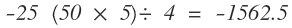1. Write number 25
2. Click on [+/-] button.
3. Click on [ * ] button –> open bracket [ ( ]  –> Type 50 –> click on [ * ] — > Type 5 –> close bracket [ ) ] –> Click on [ / ] –> Type 4 –> Click on [ = ].

Second Example: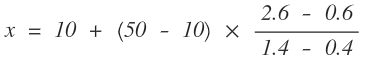Button Sequence:

10    [ + ]   [ ( ]    50    [ – ]    10    [ * ]    [ ( ]    2.6    [ – ]    0.6    [ ) ]    [ / ]     [ ( ]    1.4    [ – ]    0.4    [ ) ]    [ = ]

Power:

First we will discuss about ( power of 10 ) button then we will move toward power of other number.

Power of 10: Two button has given for Power of 10 function first is [ Exp ] and second is [ 10x ]. Let solve an example to see what is the different between them and how does they work.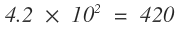while using [Exp] button we first have to type 4.2 than we will use [Exp] button but in case on [ 10x ] first we have to find power of 10 then we mention 4.2

Button Sequence:

4.2    [ Exp ]    2    [ = ]

2    [ 10x ]    [ * ]    4.2    [ = ]

Power in General

Three button has given for this purpose [ x], [ x3 ] and [ x].  First and second is for square and cube respectively where as 3rd button is for other numbers.

Square: First you have to mention x then click on [ xto get square of x. For example we want to calculate 1442 so type 144 then click on [ xfor result 20736.

Cube: First you have to mention number then click on [ x3to get cube of number. For example we want to calculate 1253 so first type number 125 then click on [ x3for result 1953125.

Other: Now we want to calculate 314. Procedure will be same as above but now we have to mention power term. So first type 31 then click on [ xnow mention power term which is 4 in this case then click on [ = ] for result 923521.

Problems: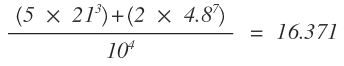Solution:

[ ( ]    [ ( ]    5    [ * ]    21    [ x]    3    [ ) ]    [ + ]    [ ( ]    2    [ * ]    4.8    [ x]    7    [ ) ]    [ ) ]    [ / ]    10    [ x]    4    [ = ].

Screenshot of virtual calculator you should get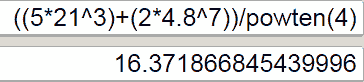Root :

Three buttons are provided for dealing with roots [ √ ],  [ 3√ ] and [ y√x ] in GATE Virtual Calculator. Let solve some simple problem to learn about these button.

Square Root: Mention the number of which square root has to be find then click on  [ √ ]. For Example we want to find √441 so first we have to type 441 then click on [ √ ] button for result 21.

Cube Root: Mention the number of which cube root has to be find then click on [ 3√ ].  For example we want to find 3√10648 so first we have to type 10648 then click on [ 3√ ] button for result 22.

Other [ y√x ]:  First type value of x then click on [ y√x ] then mention value of y. For example we want to find 5√3125 so first type 3125 then click on [ y√x ] and then mention 5 and click [ = ] for result 8.

Problem: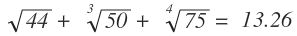Solution:

44    [ √ ]    [ + ]    50    [ 3√ ]    [ + ]    75    [ y√x ]    4     [ = ]

It should look like this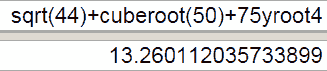Reciprocal:

For finding reciprocal of any number just type the number and click on [ 1/x ] button.

Problem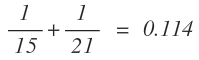Solution:

15    [ 1/x ]    [ + ]    21    [ 1/x ]    [ = ]

### Trigonometric Function

Calculator should be in degree mode and value should always be given first and then function for example in order to find sin30 one must type 30 first then click on [ sin ] button.

Gate Virtual Calculator consist of 6 buttons to deal with trigonometric problem which are [ sin ] [ cos ] [ tan ] [sin-1 ] [cos-1 ] [tan-1 and 6 hyperbolic function [ sinh ] [ cosh ] [ tanh ] [sinh-1 ] [cosh-1 ] [tanh-1 ]. Here we will look into functioning of 6 trigonometric function which will be same for hyperbolic function too.

For example:

Sin 30 + cos 30 = 1.366

30    [ sin ]    [ + ]    30    [ cos ]    [ = ]

sin230 + cos230 = 1

30    [ sin ]    [ x2 ]    [ + ]    30    [ cos ]    [ x2 ]    [ = ]

cot55 + cosec 75 = 1.735

As we know that reciprocal of sin is cosec, reciprocal of tan is cot and reciprocal of cos is sec so we will solve (1/tan55) + (1/sin75)

55    [ tan ]    [ 1/x ]    [ + ]    75    [ sin ]    [ 1/x ]    [ = ]

### Logarithmic Function

Four buttons is provided in GATE Virtual Calculator for dealing with Logarithmic Function [ ln ], [ logx ], [ log x ] and [ logx ]

[ ln ] This button mean Logarithms with base e. In order to determine ln5 first type the value which is in this case is 5 then click on [ ln ] button for result 1.609.

[ logx ] : Logarithms with base 2

[ log x ] : Logarithms with base 10

[ logx ] : Logarithms with user input base.

Problem: In mechanical, true strain of forging is given by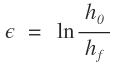Suppose value of  ho is 50 and hf is 20 then value of true strain is

Solution

50    [ / ]    20    [ = ]    [ ln ]

Ans is 0.916

### Factorial Function

we have [ n! ] button to deal with  factorial function

5!    5    [ n! ]    120

8!    8    [ n! ]    40320

1. Always click on [ C ] button before starting new calculation
2. BODMAS rule should be followed
B → Bracket
O → Order (Power and roots)
D → Division
M → Multiplication
S → Subtraction
3. Here [ mod ] button is simply a showpiece never press mod button. It is just indicating whether calculator is in deg mode or in radian mode. For changing degree mode to radian mode you have to press radio ⊙ button.
4. While solving question try to do your calculation with GATE virtual calculator in order to get good command on it.

In coming days I am going to cover calculation of important formula’s using GATE virtual Calculator for different subjects. Subscribe to get updates in your mail box.

If you want to download this tutorial in pdf form just share this post in facebook using below button. After sharing you will automatically get download link.

[sociallocker id=”8606″]

[/sociallocker]

Tags: ,

### One Response to GATE Virtual Calculator Complete Tutorial

1. anubhaw says:

Please give list of psu which are taking their own exam without gate